# Analyzing Big Data with Microsoft R v7.0

Page:    1 / 3
Exam contains 42 questions

You are running a large logistic regression for 1,000 feature variables by using the logisticRegression0 function in the MicrosoftML package. All of the predictor variables are numeric.
Currently, you specify the input variables separately by using the following formula.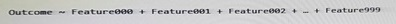You discover that it takes 20 minutes to estimate each model.
You need to reduce the amount of time required to estimate each model without losing any information in the predictors.
What should you do?

• A. Use stepControl0 to perform stepwise regression to limit the number of variables that contribute to the model.
• B. Use selectFeatures0 to select the features that provide the most information about the outcome variable.
• C. Use princomp0 on the correlation matrix of Features, and then use only the first 100 principle components to reduce the number of input variables.
• D. Use concat0 to create a single array variable named Features, and then specify a new formula named Outcome - Features.

You plan to read data from an Oracle database table and to store the data in the file system for later processing by dplyrXdf, The size of the data is larger than the memory on the server to used for modelling.
You need to ensure that the data can be processed by dplyrXdf in the least amount of time possible.
How should you transfer the data from the Oracle database?

• A. Use the RODBC library, connect to the Oracle database server by using odbcConnect. and then use rxDataStep to export the data to a comma-separated values (CSV) file. then use rxlmport to save the data to an XDF file.
• C. Use the RODBC library, connect to the Oracle database server by using odbcConnect. and then use rxSplit to save the data to multiple comma-separated values (CSV) files.

You have following regression forest.Which variable contributes the most to the dependent variable?

• A. stack.loss
• B. Water.Temp
• C. Air.Flow
• D. Acid.Conc

Note: This question Is part of a series of questions that use the same or similar answer choice. An answer choice may be correct for more than one question in the series. Each question is independent of the other questions in this series.
Information and details provided In a question apply only to that question.
You need to generate a residual based on two columns. The solution must build a trend indicator.
Which function should you use?

• A. rxPredict
• B. rxLogit
• C. Summary
• D. rxLinMod
• E. rxTweedie
• F. stepAic
• G. rxTransform
• H. rxDataStep

Note: This question is part of a series of questions that use the same scenario. For your convenience, the scenario is repeated in each question. Each question presents a different goal and answer choices, but the text of the scenario is exactly the same in each question in this series.

Start of repeated scenario -
You are developing a Microsoft R Open solution that will leverage the computing power of the database server for some of your datasets.
You are performing feature engineering and data preparation for the datasets.
The following is a sample of the dataset.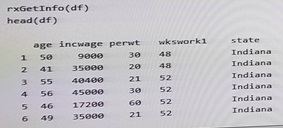End of repeated scenario -
You need to analyze the dataset without the missing values. The solution must not remove the missing values from the dataset.
Which R code segment should you use?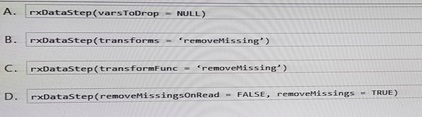• A. Option A
• B. Option B
• C. Option C
• D. Option D

Note: This question Is part of a series of questions that use the same or similar answer choice. An answer choice may be correct for more than one question in the series. Each question is independent of the other questions in this series.
Information and details provided In a question apply only to that question.
You need to evaluate the significance of coefficient that are produced by using a model that was estimated already.
Which function should you use?

• A. rxPredict
• B. rxLogit
• C. Summary
• D. rxLinMod
• E. rxTweedie
• F. stepAic
• G. rxTransform
• H. rxDataStep

Explanation: https://docs.microsoft.com/en-us/r-server/r/how-to-revoscaler-linear-model

Note: This Question is part of a series of Questions that use the same or similar answer choices. An answer choice may be correct than one question in the series.
Each question is independent of the other questions in this series. Information and details provided in a question apply only to that question.
You have a dataset that contains the physical characteristics of people.
You need to visualize a relationship between height and weight for a subset of observations in the dataset.
What should you use?

• A. the Describe package
• B. the rxHistogram function
• C. the rxSummary function
• D. the rxQuantile function
• E. the rxCube function
• F. the summary function
• G. the rxCrossTabs function
• H. the ggplot2 package

You need to set the compute context for three different target environments.
Which Statement should you use for each environment? To answer, drag the appropriate statements to the correct execution contexts. Each statement may be used once, more than once, or not at all. You may need to drag the split bar between panes or scroll to view content.
NOTE: Each correct selection is worth one point.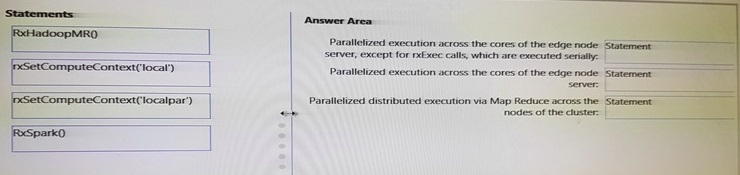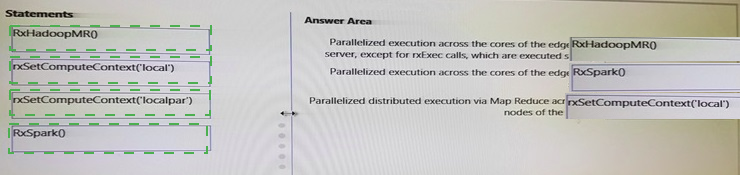You have a dataset.
You need to repeatedly split randomly the dataset so that 80 percent of the data is used as a training set and the remaining 20 percent is used as a test set.
Which method should you use?

• A. threshold
• B. binary classification
• C. imputation
• D. cross validation
• E. pruning

Note: This question is part of a series of Questions that present the same scenario. Each question in the series contains a unique solution that might meet the stated goals. Some question sets might have more than one correct solution, whale others might not have a correct solution-After you answer a question in this section, you will NOT be able to return to it- As a result, these questions will not appear in the review screen.
You use dplyrXdf. and you discover that after you exit the session, the output files that were created were deleted. You need to prevent the files from being deleted.
Solution: You use rxSetComputeContext with the local parameter before performing operations that save results.
Does this meet the goal?

• A. Yes
• B. No

Note: This question is part of a series of questions that use the same or similar answer choices. An answer choice may be correct for more than one question in the series. Each question is independent of the other questions in this series. Information and details provided in a question apply only to that question.
You need to calculate a measure of central tendency and variability for the variables in a dataset that is grouped by using another categorical variable.
What should you use?

• A. the Describe package
• B. the rxHistogram function
• C. the rxSummary function
• D. the rxQuantile function
• E. the rxCube function
• F. the summary function
• G. the rxCrossTabs function
• H. the ggplot2 package

You need to build a model that looks at the probability of an outcome. You must regulate between L1 and L2.
Which classification method should you use?

• A. Two-Class Neural Network
• B. Two-Class Support Vector Machine
• C. Two-Class Decision Forest
• D. Two-Class Logistic Regression

Note: This question is part of a series of Questions that present the same scenario. Each question in the series contains a unique solution that might meet the stated goals. Some question sets might have more than one correct solution, whale others might not have a correct solution-After you answer a question in this section, you will NOT be able to return to it- As a result, these questions will not appear in the review screen.
You use dplyrXdf and you discover that after you exit the session, the output files that were created were deleted. You need to prevent the files from being deleted.
Solution: You remove all instances of the file.remove method.
Does this meet the goal?

• A. Yes
• B. No

Note: This question Is part of a series of questions that use the same or similar answer choice. An answer choice may be correct for more than one question in the series. Each question is independent of the other questions in this series.
Information and details provided In a question apply only to that question.
You need to estimate a model where the outcome variable is continuous, is in the range of
[0,inf], and has a substantial mass at an exact value of 0.
Which function should you use?

• A. rxPredict
• B. rxLogit
• C. Summary
• D. rxLinMod
• E. rxTweedie
• F. stepAic
• G. rxTransform
• H. rxDataStep

You have a Microsoft SQI Server instance that has R Services (In Database) installed. The server has a comma separated values (CSV) file stored in the local file system.
For analytic purposes, you need to read the CSV file into a database table in the SQL
Server instance.
You connect to the SQL Server instance by using SQL Server Management Studio.
What should you use from sp_execute_external_script?

• A. RxSqIServerData and specify the CSV file path in the connecting string.
• B. rxDataStep and specify the CSV lite path as the inFile argument
• C. rxImportToXdf and specify the CSV file as the input
• D. read.csv and specify the CSV file path as the parameter.